Related Articles

# Python Pillow – Flip and Rotate Images

• Last Updated : 07 Apr, 2021

Prerequisites: Pillow

Python Pillow or PIL is the Python library that provides image editing and manipulating features. The Image Module in it provides a number of functions to flip and rotate images. image.transpose() is the function used to rotate and flip images with necessary keywords as parameters.

Syntax:

image.transpose(appropriate keyword)

In the examples given below, we will be exploring all possible rotations using an appropriate keyword.

Image used:## Flipping Images

• Anticlockwise: To flip an image in anti-clockwise direction the keyword that needs to be passed is Image.TRANSPOSE.

Syntax:

img.transpose(Image.TRANSPOSE)

Example:

## Python

 `from` `PIL ``import` `Image`` ` ` ` `img ``=` `Image.``open``(``'geek.jpg'``)`` ` `# flip anti-clockwise``flip_img ``=` `img.transpose(Image.TRANSPOSE)`` ` `flip_img.show()`

Output :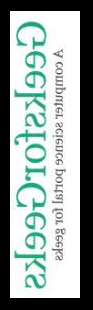Clockwise: To flip an image in the clockwise direction the keyword that needs to be passed is Image.TRANVERSE.

Syntax:

img.transpose(Image.TRANSVERSE)

Example:

## Python

 `from` `PIL ``import` `Image`` ` ` ` `img  ``=` `Image.``open``(``'geek.jpg'``)`` ` `# flip clockwise ``flip_img``=` `img.transpose(Image.TRANSVERSE)`` ` `flip_img.show()`

Output :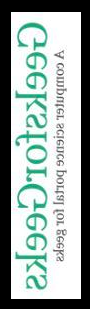Horizontal Flip: For horizontal flip, pass Image.FLIP_LEFT_RIGHT as the keyword.

Syntax :

img.transpose(Image.FLIP_LEFT_RIGHT)

Example:

## Python

 `from` `PIL ``import` `Image`` ` ` ` `img ``=` `Image.``open``(``'geek.jpg'``)`` ` `# flip horizontal``flip_img ``=` `img.transpose(Image.FLIP_LEFT_RIGHT)`` ` `flip_img.show()`

Output :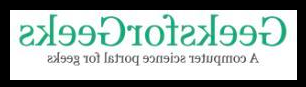Vertical flip: To flip vertically pass image.FLIP_TOP_BOTTOM as keyword

Syntax:

img.transpose(Image.FLIP_TOP_BOTTOM)

Example:

## Python

 `from` `PIL ``import` `Image`` ` ` ` `img ``=` `Image.``open``(``'geek.jpg'``)`` ` `# flip vertical``flip_img ``=` `img.transpose(Image.FLIP_TOP_BOTTOM)`` ` `flip_img.show()`

Output :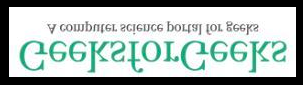## Rotating Images

Image rotation is done by specific angles and for that again specific keywords need to passed as discussed below:

Rotate by 90 degrees: The keyword used for this is Image.ROTATE_90

Syntax :

img.transpose(Image.ROTATE_90)

Example:

## Python

 `from` `PIL ``import` `Image`` ` ` ` `img ``=` `Image.``open``(``'geek.jpg'``)`` ` `# rotate by 90 degrees``rot_img ``=` `img.transpose(Image.ROTATE_90)`` ` `rot_img.show()`

Output :Rotate by 180 degrees: To rotate by 180 degrees the keyword used is Image.ROTATE_180

Syntax :

img.transpose(Image.ROTATE_180)

Example:

## Python

 `from` `PIL ``import` `Image`` ` ` ` `img ``=` `Image.``open``(``'geek.jpg'``)`` ` `# rotate by 180 degrees``rot_img ``=` `img.transpose(Image.ROTATE_180)`` ` `rot_img.show()`

Output: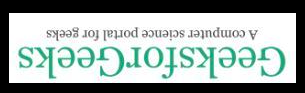Rotate by 270 degrees: To rotate by 270 degrees the keyword used is Image.ROTATE_270

Syntax :

img.transpose(Image.ROTATE_270)

Example:

## Python

 `from` `PIL ``import` `Image`` ` ` ` `img ``=` `Image.``open``(``'geek.jpg'``)`` ` `# rotate by 270 degrees``rot_img ``=` `img.transpose(Image.ROTATE_270)`` ` `rot_img.show()`

Output: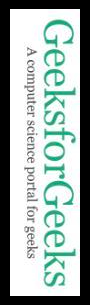Attention geek! Strengthen your foundations with the Python Programming Foundation Course and learn the basics.

To begin with, your interview preparations Enhance your Data Structures concepts with the Python DS Course. And to begin with your Machine Learning Journey, join the Machine Learning – Basic Level Course

My Personal Notes arrow_drop_up